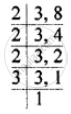# Solve: (2x)/3 - (3x)/8 = 7/12 - Mathematics

Sum

Solve: (2"x")/3 - (3"x")/8 = 7/12

#### Solution

(2"x")/3 - (3"x")/8 = 7/12L.C.M. of 3 and 8 = 2 × 2 × 2 × 3 = 24

therefore (2"x" xx 8)/(3 xx 8) - ("3x" xx 3)/(8 xx 3) = 7/12

= "16x"/24 - "9x"/24 = 7/12

= "16x"/24 - "9x"/24 = 7/12

= (16"x" - 9"x")/24 = 7/12

= "7x"/24 = 7/12

= "x" = (7 xx 24)/(12 xx 7) = 2

∴ x = 2

Concept: Solving Linear Inequations
Is there an error in this question or solution?
Chapter 14: Linear Equations in one Variable - Exercise 14 (C) [Page 169]

#### APPEARS IN

Selina Concise Mathematics Class 8 ICSE
Chapter 14 Linear Equations in one Variable
Exercise 14 (C) | Q 1.02 | Page 169
Share